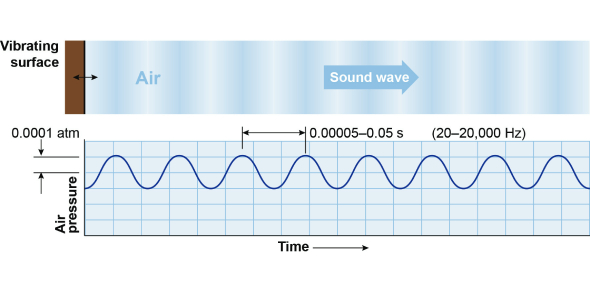# 5th Grade Sound Quiz Questions

10 Questions | Attempts: 9387
ShareSettingsAre you interested in taking a 5th-grade sound quiz? Whether you are an adult or a 5th-grade student, you can take this practice quiz. For adults, it is a memory test, and for kids, it is a practice test for upcoming exams. The quiz questions are easy enough to pass the quiz with good scores. Take the quiz and check out your scores. If you missed out on something, we would help you recall it. All the best, and try getting a perfect score.

• 1.
What is sound?
• A.

A form of loudness

• B.

A form of energy

• C.

A form of movement

• D.

A form of noise

• 2.
What causes sound?
• A.

Waves of light

• B.

Molecules moving through air

• C.

The vibration of an object

• D.

Molecules moving through space

• 3.
What unit is used to measure sound?
• A.

The decibel

• B.

The Richter scale

• C.

The wave

• D.

Metre

• 4.
What level of sound will cause immediate damage to the human ear?
• A.

200 dB

• B.

140 dB

• C.

85 dB

• D.

50 dB

• 5.
What happens to the wavelength of a sound when the frequency increases?
• A.

It decreases

• B.

It stays the same

• C.

It increases

• D.

It fluctuates

• 6.
Sound travels faster through a solid than through a gas.
• A.

True

• B.

False

• 7.
What type of waves are sound waves?
• A.

Longitudinal waves

• B.

Seismographic waves

• C.

Compressional waves

• D.

Electromagnetic waves

• 8.
Sound waves move
• A.

In the opposite direction of the source

• B.

In the same direction as the source

• C.

In all directions toward the source

• D.

In all directions away from the source

• 9.
Sound must have _____ to move through.
• A.

Velocity

• B.

Matter

• C.

Acceleration

• D.

Weight

• 10.
Sounds carry
• A.

Energy

• B.

Protons

• C.

Molecules

• D.

Electrons

## Related TopicsBack to top
×

Wait!
Here's an interesting quiz for you.